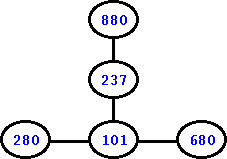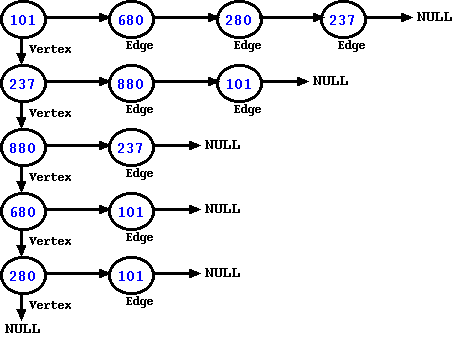CodingBison

Before we go further, let us see how a graph looks like. Well, to put it simply, we can consider a graph to be a collection of vertices and edges. We provide a simple (undirected) graph that has 5 vertices and 4 edges; each vertex is identified by a number.Figure: An undirected Graph

The adjacency list for the above graph would start with a linked list for vertices -- this list would have all the 5 vertices. Further, for each vertex, the representation would also have a linked list of all the edges that are connected to them. As an example, the vertex 280 has one edge: 280-101. So, for the vertex 280, we would see that its linked-list for edges would have one entry: 101. Please note that for an undirected graph, every edge would show up twice in the graph, one time with each vertex. Thus, 280-101 edge would mean an edge of 101 added to the vertex 280 and an edge of 280 added to the vertex 101. This is how the adjacency list would look like for the above graph.Besides undirected graphs, a graph can also additional behavior as well. The graph can also have loops. In this case, we would have edges connected to each other so that it forms a loop. In the above example, if we were to add an edge between 680 and 880 vertices, then we would have a loop. An adjacency list can accommodate graphs with loops. The graph can also have directed edges. In that case, it is not a given that one can go in either direction. In the above example, if we were to make the edge 101-680 undirected such that we can go only from 101 to 680, then the picture would have an arrow going from 101 to 680. In terms of adjacency list, we would have 680 edge-node added to vertex 101, but we would not add edge-node 101 to vertex 680.

Implementation

Let us start by providing the implementation and go over its details after that. The implementation (provided below) contains both data-structures and functions.

#include <stdio.h>
#include <stdlib.h>

typedef struct vertex_node_ {
int val;                         /* Holds an int. */
struct edge_node_   *list_enode; /* This is a linked list for all connected edges. */
struct vertex_node_ *next_vnode; /* This is the vertical linked list for all vertices. */
} vertex_node;

typedef struct edge_node_ {
void              *parent_vnode; /* Pointer to the parent vertex node. */
struct edge_node_ *next_enode;   /* This is a linked list. */
} edge_node;

/* Root of the graph */
vertex_node *graph_root = NULL;

vertex_node *new_vnode, *vnode;

vnode = graph_root;
while (vnode && vnode->next_vnode) {
/* Check, if the value already exists. */
if (vnode->val == current_value) {
return;
}
vnode = vnode->next_vnode;
}

printf("[%s] Adding Vertex Node (%d)\n", __FUNCTION__, current_value);
new_vnode = (vertex_node *)malloc(sizeof(vertex_node));
if (!new_vnode) {
return;
}
new_vnode->val = current_value;
new_vnode->list_enode = NULL;
new_vnode->next_vnode = NULL;

if (!graph_root) {
graph_root = new_vnode;
return;
}
vnode->next_vnode = new_vnode;
}

/* Add an Edge to a Vertex */
vertex_node *second_vnode,
int val_second_vertex) {
edge_node *enode, *prev_enode, *new_enode;

if (!first_vnode || !second_vnode)
return;

prev_enode = enode = first_vnode->list_enode;
while (enode) {
if (((vertex_node *)enode->parent_vnode)->val == val_second_vertex) {
return;
}
prev_enode = enode;
enode = enode->next_enode;
}

new_enode = (edge_node *)malloc(sizeof(edge_node));
if (!new_enode) {
fprintf(stderr, "[%s] Malloc failed \n", __FUNCTION__);
return;
}
new_enode->parent_vnode = second_vnode;
new_enode->next_enode = NULL;
if (prev_enode) {
prev_enode->next_enode = (void *)new_enode;
} else {
/* prev_enode is NULL means new_enode is the first enode for this vertex */
first_vnode->list_enode = (void *)new_enode;
}
}

vertex_node *vertex_nodeA = NULL, *vertex_nodeB = NULL, *vnode;

/*
* First, find the vnode for both vertices in the edge. We will
* add an enode to both of these vnodes.
*/
for (vnode = graph_root; vnode != NULL; vnode = vnode->next_vnode) {
if (vnode->val == vertexA) {
vertex_nodeA = vnode;
}
if (vnode->val == vertexB) {
vertex_nodeB = vnode;
}
if ((vertex_nodeA) && (vertex_nodeB)) {
break;
}
}

if (!vertex_nodeA) {
return;
}

if (!vertex_nodeB) {
return;
}

/* Add Edge to VertexA. When added, this edge would point to VertexB */
printf("\t[%s] Adding Edge (%d-%d) to Vertex Node (%d) \n",
__FUNCTION__, vertexA, vertexB, vertexA);

/* Add Edge to VertexB. When added, this edge would point to VertexA */
printf("\t[%s] Adding Edge (%d-%d) to Vertex Node (%d) \n",
__FUNCTION__, vertexA, vertexB, vertexB);
}

/* Free the Adjacency List */
vertex_node *vnode = NULL, *prev_vnode = NULL;
edge_node *enode = NULL, *prev_enode = NULL;

vnode = graph_root;
/* Walk the list of vertex nodes. */
while (vnode) {
/* For each vertex node, walk the list of edge nodes. */
enode = vnode->list_enode;
while (enode) {
prev_enode = enode;
enode = enode->next_enode;
free(prev_enode);
}
prev_vnode = vnode;
vnode = vnode->next_vnode;
free(prev_vnode);
}
graph_root = NULL;
}

/* Print the Adjacency List */
vertex_node *vnode = NULL;
edge_node *enode = NULL;

printf("[%s] Print the Adjacency List", __FUNCTION__);
if (!graph_root) {
printf("\n[%s] Nothing to print. Return\n", __FUNCTION__);
return;
}

for (vnode = graph_root; vnode != NULL; vnode = vnode->next_vnode) {
printf("\n\tVertex [%3d]:", vnode->val);
for (enode = vnode->list_enode; enode != NULL; enode = enode->next_enode) {
printf(" -- Edge [%3d]", ((vertex_node *)enode->parent_vnode)->val);
}
}
printf("\n");
}

int main () {
int vertices[] = {101, 237, 880, 680, 280};
int edges[] = {{237, 880}, {101, 680}, {101, 280}, {237, 101}};
int len = sizeof(vertices)/sizeof(vertices);
int i;

for (i = 0; i < len; i++) {
}

len = sizeof(edges)/sizeof(edges);
for (i = 0; i < len; i++) {
}

/* Done with the Adjacency List. Free it*/
}

Let us first start with data-structures. The above file has two data structures: vertex_node for vertices and edge_node for edges. The vertex_node data-structures supports two linked lists -- one for the vertices and one for the edges belonging to a given vertex. The next_vnode pointer enables linked list for vertices and all the vertices of a graph are added to this linked list. The list_enode pointer enables linked list for all edges that belong to a given vertex.

Besides the linked list pointer (next_enode), the edge_node also contains one additional members. The "parent_vnode" pointer holds the the address of the vertex node that is added to the edge list. Thus, if an edge is formed by vertices A-B, then when we add the edge to the vertex A, the parent_vnode of the edge would point to the vertex B. Likewise, when we add the edge A-B to the vertex B, the parent_vnode of the edge would point to the vertex A. Depending upon the use-case, it is possible to simplify this where the edge node contains the value (identifier) of the vertex. However, keeping a pointer allows us to have the edge node access the entire data associated with the vertex node and for cases, where we need to store more than an integer (the "val" member), a pointer would be more efficient.

Next, let us go over these functions.

The adjlist_add_vertex() is the function that adds a vertex to the adjacency list. It starts by walking the vertices linked list. If it finds an existing vertex with the same identifier (value), then it bails out. If not, then it walks to last vnode of the list. If the vertex is not found, then it mallocs a new vertex node and adds it to the last node.

The adjlist_print() and adjlist_free() are in some ways traversal routines. They walk over each vertex and edge of the graph. The adjlist_free() function that frees up all vertices and edges belonging to the graph. The adjlist_print() prints all the vertices and edges belonging to the graph -- we can use this to dump all edges and vertices belonging to a graph.

In the end, we have the main function that provides a simple user-case of the adjacency list implementation. From this function, we invoke above functions to add vertices, add edges, print the adjacency list, and then in the end, free the graph. Here is the compilation step and the output.

Vertex :
Vertex :
Vertex :
Vertex :
Vertex :

Vertex : -- Edge  -- Edge  -- Edge 
Vertex : -- Edge  -- Edge 
Vertex : -- Edge 
Vertex : -- Edge 
Vertex : -- Edge 

Due to sake of simplicity, we have kept the above implementation (intentionally) simple. Let us now give some thoughts as to how we can make the above implementation better.

First, we can certainly make the above code more modular. Instead of having one monolithic file, we can split it into three files: (a) a header file that publishes the data-structures and the APIs, (b) a C file that contains the implementation of the published APIs, and (c) another C file that contains the main() routine and uses the adjacency list implementation. When we use this implementation for various applications, having the implementation in three different files allows applications to reuse the header file and the implementation file.

Second, the above implementation has the graph_root as a global variable. This will work fine, if we keep this in one single file. However, when we have multiple applications reusing the same implementation, then keeping graph_root as global would not work. In that case, we must allow each application to define and use their own graph_root variable.

Third, for a generic application, we would probably need to add more information for both vertex and edges nodes. For vertex nodes, we could add information like node color, some node-specific data, etc. For edges nodes, we could add information like weight of the edge, etc.

If you would like to see an implementation that follows the above recommendation to make the code more generic, then you can find the complete program here: Advanced Implementation of Adjacency List.